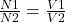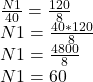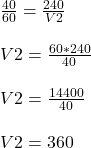## You are fixing a transformer for a toy truck that uses an 8.0-V emf to run it. The primary coil of the transformer is broken; the secondary

Question

You are fixing a transformer for a toy truck that uses an 8.0-V emf to run it. The primary coil of the transformer is broken; the secondary coil has 40 turns. The primary coil is connected to a 120-V wall outlet.
(a) How many turns should you have in the primary coil?
(b) If you then connect this primary coil to a 240-V source, what emf would be across the secondary coil?
Comments: The relevant equation is N1/N2 = V1/V2 where N is the number of turns and V is the voltage. I’m just not sure how to get the voltage of the secondary coil using emf.

in progress 0
3 months 2021-08-13T20:26:32+00:00 1 Answers 0 views 0

a. The primary turns is 60 turns

b. The secondary voltage will be 360 volts.

Explanation:

Given data

secondary turns N2= 40 turns

primary turns N1= ?

primary voltage V1= 120 volts

secondary voltage V2= 8 volts

Applying the transformer formula which iswe can solve for N1 by substituting into the equation abovethe primary turns is 60 turns

If the primary voltage is V1 240 volts hence the secondary voltage V2 will be (to get the voltage of the secondary coil using emf substitute the values of the previously gotten N1 and N2 using V1 as 240 volts)the secondary voltage will be 360 volts.